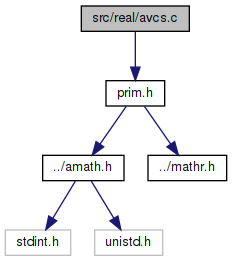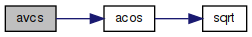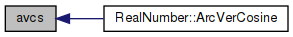amath  1.8.5 Simple command line calculator
avcs.c File Reference

Inverse versed sine. More...

`#include "prim.h"`
Include dependency graph for avcs.c:Go to the source code of this file.

## Functions

double avcs (double x)
Inverse versed sine. More...

## Detailed Description

Inverse versed sine.

Definition in file avcs.c.

## ◆ avcs()

 double avcs ( double x )

Inverse versed sine.

```avcs(x) = acos(1+x)
```

Definition at line 44 of file avcs.c.

References acos().

Referenced by RealNumber::ArcVerCosine().

45 {
46  return acos(1.0 + x);
47 }
double acos(double x)
Inverse cosine function.
Definition: acos.c:92
Here is the call graph for this function:Here is the caller graph for this function: